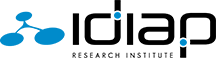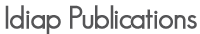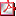[BibTeX] [Marc21]
Geometric Algebra for Optimal Control with Applications in Manipulation Tasks
 Type of publication: Journal paper Citation: Low_T-RO-2023 Publication status: Accepted Journal: IEEE Transactions on Robotics Year: 2023 Month: May Abstract: Many problems in robotics are fundamentally problems of geometry, which lead to an increased research effort in geometric methods for robotics in recent years. The results were algorithms using the various frameworks of screw theory, Lie algebra and dual quaternions. A unification and generalization of these popular formalisms can be found in geometric algebra. The aim of this paper is to showcase the capabilities of geometric algebra when applied to robot manipulation tasks. In particular the modelling of cost functions for optimal control can be done uniformly across different geometric primitives leading to a low symbolic complexity of the resulting expressions and a geometric intuitiveness. We demonstrate the usefulness, simplicity and computational efficiency of geometric algebra in several experiments using a Franka Emika robot. The presented algorithms were implemented in c++20 and resulted in the publicly available library gafro. The benchmark shows faster computation of the kinematics than state-of-the-art robotics libraries. Keywords: Projects Authors Added by: [UNK] Total mark: 0 AttachmentsLow_T-RO-2023.pdf Notes